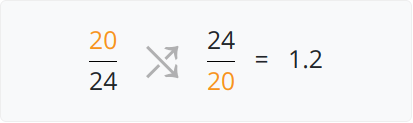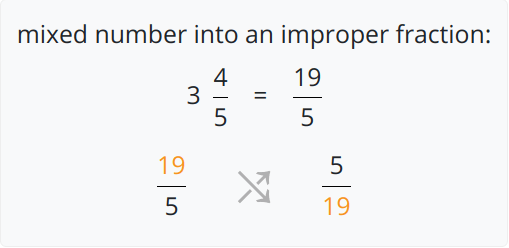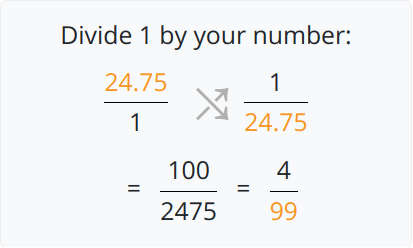# Reciprocal Calculator

To find the reciprocal, select the input type, enter the values, and click calculate button using reciprocal calculatorReciprocal of a fraction calculator is used to find the reciprocal form of different kinds of fractions and numbers i.e decimals, integers, proper, and mixed number fractions.

It finds the reciprocal of integers and decimals with a step-wise guide. Click the loading icon to try this calculator for few examples.

## What is a reciprocal?

A reciprocal can be defined as the inverse of a fraction or number. Technically, it inverses the sign of a number.

For example: If a number is 32, its reciprocal will be -32.

## Reciprocal formula

There isn’t any special formula to find the reciprocal of a number. You just use a technique that swaps the denominator and the numerator.

Alternatively, you can divide the number by 1.

## How to find the reciprocal?

### Example:

• Of a simple fraction:

Swap the numerator with the denominator.• Of a mixed fraction:

Solve the mixed fraction to a proper fraction.• Of an Integer/decimal:

Divide by 1 and convert the decimal to fraction.